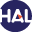# Volume 2 N°1 (2005)

Latest addition : 29 December 2010.

• ### On the numerical simulation of multiphase water flows with changes of phase and strong gradients using the Homogeneous Equilibrium Model

4 January 2005,

Full Title: On the numerical simulation of multiphase water flows with changes of phase and strong gradients using the Homogeneous Equilibrium Model

Abstract:

We introduce a general method based on a variant of the Flux Characteristic method described by Ghidaglia et al  designed to simulate water-vapour two-phase flows. As an example, we use the three equations Homogeneous Equilibrium model (HEM) with hypotheses of local thermodynamic equilibrium. Our purpose here is to analyze the Finite Volume method when strong gradients of density are present and when some derivatives of quantities like speed of sound strongly vary due to phase transitions. As framework for numerical experiments, we consider a complex flow inside an injector-condenser device. The analysis will lead to a variant of the Flux Characteristic method with regularized matrix-valued sign functions. Other applications like water boiling into a hot channel and a fall of pressure in a crack due to friction will be also considered.

Date of publication : January 2005Voir sur HAL : https://hal.archives-ouvertes.fr/hal-01123347

• ### A staggered finite volume scheme on general meshes for the Navier-Stokes equations in two space dimensions

4 January 2005,

Full Title: A staggered finite volume scheme on general meshes for the Navier-Stokes equations in two space dimensions

Abstract: This paper presents a new finite volume scheme for the incompressible steady-state Navier-Stokes equations on a general 2D mesh. The scheme is staggered, i.e. the discrete velocities are not located at the same place as the discrete pressures. We prove the existence and the uniqueness of a discrete solution for a centered scheme under a condition on the data, and the unconditional existence of a discrete solution for an upstream weighting scheme. In both cases (nonlinear centered and upstream weighting schemes), we prove the convergence of a penalized version of the scheme to a weak solution of the problem. Numerical experiments show the efficiency of the schemes on various meshes.

Date of publication: January 2005

Presented by Professor LazarovVoir sur HAL : https://hal.archives-ouvertes.fr/hal-01112401

• ### A staggered finite volume scheme on general meshes for the generalized Stokes problem in two space dimensions

4 January 2005,

Description: Authors:

Ph. Blanc, R. Eymard and R. Herbin

Full Title:

A staggered finite volume scheme on general meshes for the generalized Stokes problem in two space dimensions

Abstract:

This paper presents a new ﬁnite volume scheme for the steady Stokes equations on a general 2D mesh. The scheme is staggered, i.e. the discrete velocities are not located at the same place as the discrete pressures. We prove the existence and the uniqueness of a discrete solution.

We then prove convergence of the discrete velocities to the weak solution of the problem. Under additional regularity conditions, we prove the convergence of a penalized version of the scheme to the weak solution of the problem. Numerical experiments on problems with known analytical solutions allow to obtain the rate of convergence for both velocities and pressure.

Date of publication : January 2005

Paper presented by professor LazarovVoir sur HAL : https://hal.archives-ouvertes.fr/hal-01112399